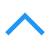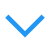# 目标检测评价指标(mAP)

## 常见指标

• precision 预测出的所有目标中正确的比例 (true positives / true positives + false positives).
• recall 被正确定位识别的目标占总的目标数量的比例 (true positives/(true positives + false negatives)).

Precision和Recall的计算图示如下: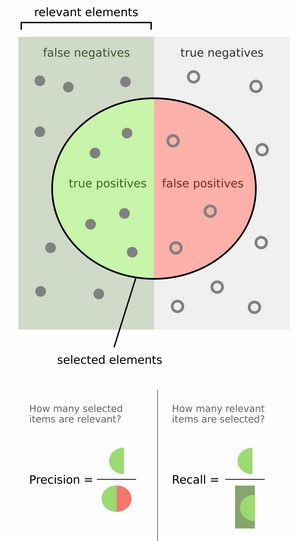• F1 score 通常我们使用precision和recall两个指标来衡量模型的好坏,但是同时要权衡这两个量,影响我们做决策的速度.可以使用F1 score来组合这两个量(又称F score,F measure,名称F没有什么意义):

$F_1\ \text{Score} ={2\over {1\over P}+{1\over R}}= 2{PR\over P+R}\in[0,1]$

F1 score 是 $F=1/(\lambda{1\over P}+(1-\lambda){1\over R})$ 的简化版($\lambda=0.5$).
P和R的值均是越大越好,因此F1 score也越大结果越好.

• AUC ROC 曲线下的面积,面积越大,分类效果越好.ROC横轴为假正率(FP,false positive),纵轴为真正率(TP,true positive)。 通过给分类器设置不同的置信度阈值得到多组（FP,TP）数据绘制成ROC 曲线。ROC 曲线如下图所示：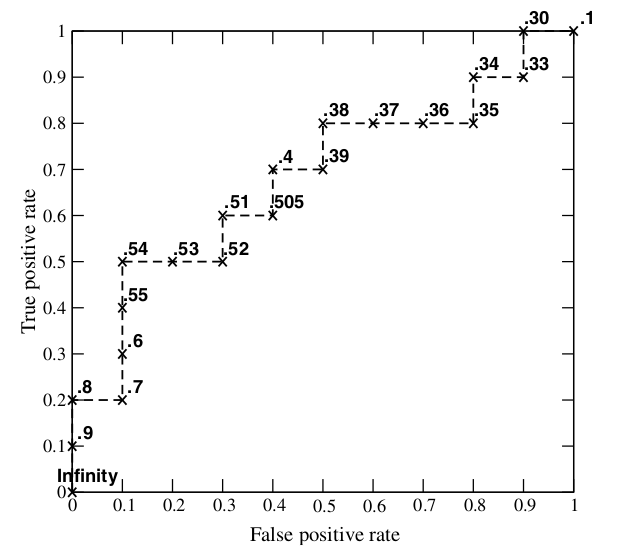AUC 的含义：AUC值是一个概率值，当你随机挑选一个正样本以及一个负样本，当前的分类算法根据计算得到的Score值将这个正样本排在负样本前面的概率就是AUC值。当然，AUC值越大，当前的分类算法越有可能将正样本排在负样本前面，即能够更好的分类。

• loss_bbox 预测边框和真实边框的坐标之间的差别,如采用smooth L1 loss计算.
• mAP 对于每一类计算平均精度(AP,average precision),然后计算所有类的均值。mAP 综合考量了P、R，解决P，R的单点值局限性。PR曲线与ROC曲线类似，曲线下面积越大越好，因此我们定义PR曲线下面积为：

$\rm{mAP} = \int_0^1 P(R)dR$

## mAP (VOC)

$AP = {1\over 11}\sum_{r\in \{0,0.1,...,1\}} P_{interp}(r) \\ \text{mAP} = {\sum_{q=1}^Q AP(q)\over Q}$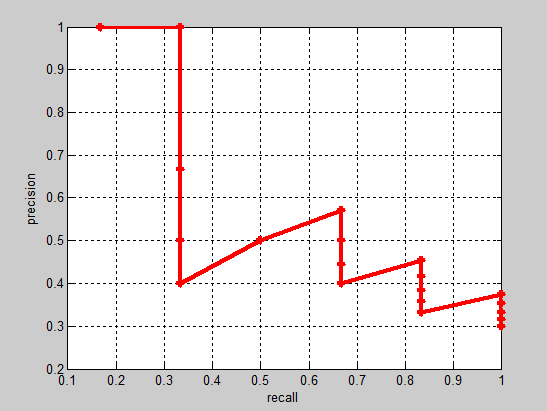1. 对于类别C，首先将算法输出的所有C类别的预测框，按置信度排序；
2. 设定不同的k值，选择top k个预测框，计算FP和TP，使得 recall 分别等于0，0.1，0.2，0.3，0.4，0.5，0.6，0.7，0.8，0.9，1.0；
计算Precision；
3. 将得到的11个Precision取平均，即得到AP；
AP是针对单一类别的，mAP是将所有类别的AP求和，再取平均：
mAP = 所有类别的AP之和 / 类别的总个数

PASCAL VOC challenge 自2010年后换了另一种计算方法,提高了计算的精度,能更好地区分低准确率的方法。新的计算方法假设这N个样本中有M个正例，那么我们会得到M个recall值,对于每个recall值r，我们可以计算出对应（r' > r）的最大precision，然后对这M个precision值取平均即得到最后的AP值。参考voc2012/devkit_doc.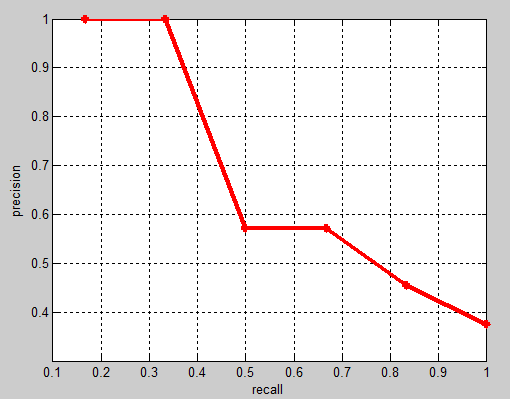AP衡量的是学出来的模型在每个类别上的好坏，mAP是取所有类别AP的平均值，衡量的是在所有类别上的平均好坏程度。

## AP(COCO)

mAP@[.5:.95]是在不同 IoU (从 0.5 到 0.95, 步长0.05) 设置下的平均值,又可写作mmAP或 AP, 参考cocoeval.py.

## mAP 代码参考

VOC 目标检测评估函数

function [rec,prec,ap] = VOCevaldet(VOCopts,id,cls,draw)

% npos 为ground truth objects的数目, 计算中会忽略difficult的样本
% sort detections by decreasing confidence
[sc,si]=sort(-confidence);   %按照score降序排序
ids=ids(si);
BB=BB(:,si);

% assign detections to ground truth objects
nd=length(confidence);
tp=zeros(nd,1);
fp=zeros(nd,1);
tic;
for d=1:nd
% find ground truth image
i=strmatch(ids{d},gtids,'exact');
if isempty(i)
error('unrecognized image "%s"',ids{d});
elseif length(i)>1
error('multiple image "%s"',ids{d});
end

% assign detection to ground truth object if any
bb=BB(:,d);
ovmax=-inf;
for j=1:size(gt(i).BB,2)
bbgt=gt(i).BB(:,j);
bi=[max(bb(1),bbgt(1)) ; max(bb(2),bbgt(2)) ; min(bb(3),bbgt(3)) ; min(bb(4),bbgt(4))];
iw=bi(3)-bi(1)+1;
ih=bi(4)-bi(2)+1;
if iw>0 & ih>0
% compute overlap as area of intersection / area of union
ua=(bb(3)-bb(1)+1)*(bb(4)-bb(2)+1) + (bbgt(3)-bbgt(1)+1)*(bbgt(4)-bbgt(2)+1) - iw*ih;
ov=iw*ih/ua;
if ov>ovmax
ovmax=ov;
jmax=j;
end
end
end
% assign detection as true positive/don't care/false positive
if ovmax>=VOCopts.minoverlap
if ~gt(i).diff(jmax)
if ~gt(i).det(jmax)
tp(d)=1;            % true positive
gt(i).det(jmax)=true;
else
fp(d)=1;            % false positive (multiple detection)   % 若多个目标框对应同一个gt，则将后续(低score的)目标设为FP
end
end
else
fp(d)=1;                    % false positive
end
end

% 由于目标框按score排序,设置不同的score阈值可以得到不同的P/R, 因此可以通过累加操作计算
fp=cumsum(fp);
tp=cumsum(tp);
rec=tp/npos;
prec=tp./(fp+tp);

% VOC2007: compute 11 point average precision
ap=0;
for t=0:0.1:1
p=max(prec(rec>=t));
if isempty(p)
p=0;
end
ap=ap+p/11;
end

% VOC2012:
ap_new = VOCap(rec,prec);

% VOCap计算方式如下:
function ap = VOCap(rec,prec)

mrec=[0 ; rec ; 1]; % 在召回率列表首尾添加两个值
mpre=[0 ; prec ; 0];
for i=numel(mpre)-1:-1:1
mpre(i)=max(mpre(i),mpre(i+1)); % 使mpre单调递减
end
i=find(mrec(2:end)~=mrec(1:end-1))+1; % 找出召回率产生变化的下标
ap=sum((mrec(i)-mrec(i-1)).*mpre(i)); % 计算ROC曲线下面积



## 重叠度（IoU,Intersect over Union）: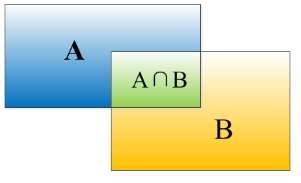IoU就是矩形框A、B的重叠面积占A、B并集的面积比例($A\bigcap B\over A\bigcup B$)。

• Correct: 类别正确 且 IoU > .5
• Localization: 类别正确, .1 < IoU < .5
• Similar: 类别近似, IoU > .1
• Other: 类别错误, IoU > .1
• Background: IoU < .1 的任意目标

python代码实现

# Calculate Intersect over usion between boxes b1 and b2, here each box is defined with 2 points
# box(startX, startY, endX, endY), there are other definitions ie box(x,y,width,height)
def calc_iou(b1, b2):
# determine the (x, y)-coordinates of the intersection rectangle
xA = max(b1, b2)
yA = max(b1, b2)
xB = min(b1, b2)
yB = min(b1, b2)

# compute the area of intersection rectangle
area_intersect = (xB - xA + 1) * (yB - yA + 1)

# Calculate area of boxes
area_b1 = (b1 - b1 + 1) * (b1 - b1 + 1)
area_b2 = (b2 - b2 + 1) * (b2 - b2 + 1)

# compute the intersection over union by taking the intersection
# area and dividing it by the sum of prediction + ground-truth
# areas - the intersection area
iou = area_intersect / float(area_b1 + area_b2 - area_intersect)

# return the intersection over union value
return iou

# IoU 实现 numpy 方式
import numpy as np

def calc_iou_np(xy_min1, xy_max1, xy_min2, xy_max2):
# Get areas
areas_1 = np.multiply.reduce(xy_max1 - xy_min1)
areas_2 = np.multiply.reduce(xy_max2 - xy_min2)

# determine the (x, y)-coordinates of the intersection rectangle
_xy_min = np.maximum(xy_min1, xy_min2)
_xy_max = np.minimum(xy_max1, xy_max2)
_wh = np.maximum(_xy_max - _xy_min, 0)

# compute the area of intersection rectangle
_areas = np.multiply.reduce(_wh)

# return the intersection over union value
return _areas / np.maximum(areas_1 + areas_2 - _areas, 1e-10)


## 参考

posted @ 2018-04-02 21:15  康行天下  阅读(26889)  评论(4编辑  收藏  举报